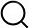# Also in the Article

Critical points

Constructing graphs from genetic encodings
Sci Rep, Jun 24, 2021;

Procedure

In this section we propose approximations for the transitions from subcritical to supercritical to connected phases of the RG model. We consider the critical point of the system to be equivalent to an ER graph’s critical point, when $⟨k⟩=1$. The density of the system was derived in-text, and multiplying by the number of nodes we can write:

where $π=2x-b$. We found it useful to solve for $Rcrit(x,b)$ which is the number of rules introduced to reach the critical point for a given x and b:

Alternatively, we consider the transition from supercritical to connected regime to occur when the nodes are ‘covered’ by wiring rules, by which we mean that there are sufficient rules for every node to participate in at least 1 rule if there were no overlap. In this manner, $Rcrit(x,b)$ can be found as the number of nodes divided by the number of nodes in a wiring rule:

Q&A